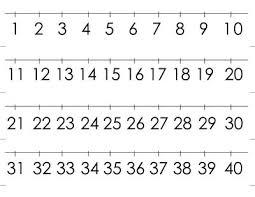# Equations 5445

The digit sum of a two-digit number is 8. If we change the order of the digits, we get a number 18 smaller than the original. Identify these numbers. We are using linear equations of two unknowns.

a =  53
b =  35

### Step-by-step explanation:

a = x·10 + y
b = y·10+x
x+y = 8
b = a - 18

a-10x-y = 0
b-x-10y = 0
x+y = 8
a-b = 18

Row 4 - Row 1 → Row 4
a-10x-y = 0
b-x-10y = 0
x+y = 8
-b+10x+y = 18

Row 4 + Row 2 → Row 4
a-10x-y = 0
b-x-10y = 0
x+y = 8
9x-9y = 18

Pivot: Row 3 ↔ Row 4
a-10x-y = 0
b-x-10y = 0
9x-9y = 18
x+y = 8

Row 4 - 1/9 · Row 3 → Row 4
a-10x-y = 0
b-x-10y = 0
9x-9y = 18
2y = 6

y = 6/2 = 3
x = 18+9y/9 = 18+9 · 3/9 = 5
b = 0+x+10y = 0+5+10 · 3 = 35
a = 0+10x+y = 0+10 · 5+3 = 53

a = 53
b = 35
x = 5
y = 3

Our linear equations calculator calculates it.Did you find an error or inaccuracy? Feel free to write us. Thank you!

Tips for related online calculators
Do you have a system of equations and looking for calculator system of linear equations?